# Test: Networks & Control Systems- 1

## 15 Questions MCQ Test GATE Electrical Engineering (EE) 2023 Mock Test Series | Test: Networks & Control Systems- 1

Description
Attempt Test: Networks & Control Systems- 1 | 15 questions in 45 minutes | Mock test for GATE preparation | Free important questions MCQ to study GATE Electrical Engineering (EE) 2023 Mock Test Series for GATE Exam | Download free PDF with solutions
QUESTION: 1

Solution:
QUESTION: 2

Solution:
QUESTION: 3

### The reactance offered by a capacitor to alternating current of frequency 50 Hz is 20 Q. If frequency is increased to 100 Hz, reactance becomes_____ohms.

Solution:
QUESTION: 4

Given the current through R is i = 1A. The value of R is ______________ Ω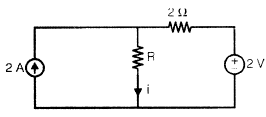Solution:
QUESTION: 5

A series RLC circuit has bandwidth as 1000 Hz with R = 10 Ω. If the resistance ‘R’ increased to 20 Ω, then the bandwidth of  the circuit is __________

Solution:
QUESTION: 6

In a series RL circuit, 12 V rms is measured across the resistor, and 14 V rms is measured across the inductor. The peak value of the source voltage is

Solution:
QUESTION: 7

Current in some branches are given in the below figure. The value of T is ____________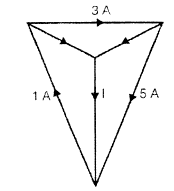Solution:
QUESTION: 8

The circuit shown below is the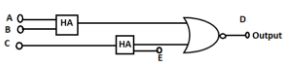Solution:
QUESTION: 9

A system has bandwidth as 4000 Hz with rise time 2.5 seconds. If the rise time decreased to 1.25 seconds then the bandwidth is ___________ Hz

Solution:
QUESTION: 10

A system with pole at -1 +j had settling time as 3 secs. If the pole shifted to -2+3j the settling time is _________ sec

Solution:
QUESTION: 11

In “force - current” analogy, equivalent of viscous coefficient is _________ and in “force - voltage" analogy, equivalent of spring constant is ______________

Solution:
QUESTION: 12

A system has transfer function (5 - S)/(5 + S). It is a _______

Solution:
QUESTION: 13

Consider the following ‘3’ transfer functions.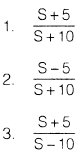Among these minimum phase system (s) i s/are

Solution:
QUESTION: 14

The purpose of Pi controller is to improve ___________ of the system.

Solution:
QUESTION: 15

Below figure is a pole-zero plot of a ____________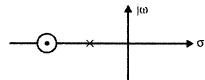Solution:Use Code STAYHOME200 and get INR 200 additional OFF Use Coupon Code